### Qin Shi Haung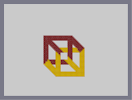Hover over the thumbnail for a full-size version.

Author quegurjin author:quegurjin fun-to-look-at n-art nonplayable optical rated 2008-12-16 2008-12-16 4 by 5 people. \$Qin Shi Haung#quegurjin#none#00000000000000000000000000000000000000000000000000000000000000000000000000000000000000000000000000000000000000000000000000000000000000000000000000000000000000000000000000000000000000000000000000000000000000000000000000000000000000000000000000000000000000000000000000000000000000000000000000000000000000000000000000000000000000000000000000000000000000000000000000000000000000000000000000000000000000000000000000000000000000000000000000000000000000000000000000000000000000000000000000000000000000000000000000000000000000000000000000000000000000000000000000000000000000000000000000000000000000000000000000000000000000000000000000000000000000000000000000000000000000000000000000000000000000000000000000000000000000000|0^348,324!0^372,300!0^396,300!0^444,300!0^492,324!0^492,348!0^492,372!0^492,396!0^492,420!0^492,444!0^468,444!0^444,444!0^420,444!0^396,444!0^372,444!0^348,444!0^348,420!0^348,396!0^348,372!0^348,348!12^420,348!12^420,324!12^420,300!12^420,276!12^420,252!12^396,372!12^372,372!12^324,372!12^276,348!12^420,228!12^396,228!12^372,228!12^348,228!12^324,228!12^300,228!12^276,228!12^276,252!12^276,276!12^276,300!12^276,324!12^420,312!12^420,336!12^384,372!12^312,372!12^276,360!12^276,336!12^276,312!12^276,288!12^276,264!12^276,240!12^288,228!12^312,228!12^336,228!12^360,228!12^384,228!12^408,228!12^420,240!12^420,264!12^420,288!12^432,300!12^432,288!12^432,276!12^432,264!12^432,252!12^432,240!12^432,228!12^432,216!12^420,216!12^408,216!12^396,216!12^384,216!12^372,216!12^348,216!12^360,216!12^336,216!12^324,216!12^312,216!12^300,216!12^276,216!12^288,216!12^264,216!12^264,228!12^264,240!12^264,252!12^264,264!12^264,276!12^264,288!12^264,300!12^264,312!12^264,324!12^264,336!12^264,348!12^264,360!12^324,384!12^372,384!12^384,384!12^396,384!12^408,384!12^432,360!12^432,336!12^432,348!12^432,324!12^432,312!12^408,336!12^408,324!12^408,312!12^408,300!12^408,276!12^408,288!12^408,264!12^408,252!12^408,240!12^396,240!12^384,240!12^372,240!12^360,240!12^348,240!12^336,240!12^312,240!12^324,240!12^300,240!12^288,240!12^288,252!12^288,264!12^288,276!12^288,288!12^288,312!12^288,300!12^288,324!12^288,348!12^288,336!12^300,360!12^312,360!12^324,360!12^372,360!12^384,360!0^348,360!0^348,384!0^348,408!0^348,432!0^360,444!0^384,444!0^408,444!0^432,444!0^456,444!0^480,444!0^492,432!0^492,408!0^492,384!0^492,360!0^492,336!0^492,312!0^384,300!0^348,336!0^396,312!0^384,312!0^360,336!0^360,348!0^360,360!0^360,372!0^360,384!0^336,384!0^336,360!0^336,372!0^336,348!0^336,336!0^336,324!0^336,312!0^372,288!0^384,288!0^360,288!0^396,288!0^360,396!0^360,408!0^360,420!0^360,432!0^372,432!0^384,432!0^408,432!0^396,432!0^432,432!0^420,432!0^444,432!0^456,432!0^480,432!0^468,432!0^480,420!0^480,408!0^480,396!0^480,384!0^480,372!0^480,360!0^480,336!0^480,348!0^480,324!0^468,312!0^456,312!0^444,312!0^444,288!0^504,312!0^504,324!0^504,348!0^504,336!0^504,360!0^504,384!0^504,372!0^504,396!0^504,408!0^504,432!0^504,420!0^504,444!0^504,456!0^492,456!0^480,456!0^468,456!0^444,456!0^456,456!0^432,456!0^408,456!0^420,456!0^396,456!0^384,456!0^372,456!0^348,456!0^360,456!0^336,456!0^336,444!0^336,432!0^336,420!0^336,396!0^336,408!0^408,348!0^408,360!0^396,360!0^408,372!0^420,384!0^420,372!0^420,360!0^432,372!0^432,384!0^432,396!0^444,408!0^456,420!0^468,420!0^468,408!0^456,408!0^456,396!0^444,396!0^444,384!12^360,324!12^360,312!12^372,312!12^360,300!12^348,300!12^348,312!12^348,288!12^336,300!12^336,288!12^336,276!12^324,288!12^324,276!12^324,264!12^312,276!12^312,264!12^312,252!12^300,264!12^300,252!0^504,300!12^264,372!12^480,276!12^468,276!12^492,300!12^480,300!12^468,288!12^480,288!12^492,288!12^492,276!12^480,264!12^468,264!12^468,252!12^456,252!12^456,240!12^444,240!12^444,228!12^456,276!12^456,264!12^444,264!12^444,252!0^456,300!0^456,288!12^504,288!12^312,384!12^300,372!0^288,372!0^276,372!0^264,384!0^288,384!0^276,396!0^276,384!0^324,444!0^312,432!0^300,420!0^288,408!0^300,396!0^288,396!0^300,384!0^312,408!0^300,408!0^312,420!0^324,432!0^324,420!0^324,408!0^312,396!0^288,360!0^498,456!0^498,450!0^504,450!0^498,444!0^504,438!0^504,426!0^504,414!0^504,402!0^504,390!0^504,378!0^504,366!0^504,354!0^504,342!0^504,330!0^504,318!0^504,306!0^498,312!0^486,324!0^492,318!0^498,318!0^498,330!0^498,324!0^498,342!0^498,336!0^498,348!0^498,360!0^498,354!0^498,366!0^498,372!0^498,384!0^498,378!0^498,390!0^498,396!0^498,402!0^498,414!0^498,420!0^498,432!0^498,438!0^498,426!0^498,408!0^480,330!0^480,342!0^486,336!0^486,348!0^492,342!0^492,330!0^486,330!0^486,342!0^486,354!0^486,360!0^486,372!0^486,366!0^486,378!0^486,384!0^486,390!0^486,396!0^486,408!0^486,402!0^486,414!0^486,426!0^486,420!0^486,456!0^474,456!0^486,450!0^486,444!0^486,438!0^486,432!0^492,450!0^492,438!0^492,426!0^492,414!0^492,402!0^492,390!0^492,378!0^492,366!0^492,354!0^480,354!0^480,366!0^480,378!0^480,390!0^480,402!0^480,414!0^480,426!0^480,438!0^480,450!0^474,450!0^474,444!0^474,438!0^474,432!0^474,426!0^474,420!0^474,414!0^462,414!0^468,414!0^462,420!0^462,426!0^468,426!0^468,438!0^462,438!0^462,444!0^462,450!0^468,450!0^462,456!0^462,432!0^456,414!0^450,414!0^438,402!0^426,390!0^414,378!0^402,366!0^408,354!0^396,360!0^414,354!0^426,366!0^438,378!0^450,390!0^462,402!0^462,408!0^450,408!0^456,402!0^450,402!0^444,402!0^450,396!0^438,396!0^444,390!0^438,390!0^438,384!0^432,390!0^426,378!0^426,384!0^432,378!0^420,378!0^426,372!0^414,372!0^414,366!0^408,366!0^420,366!0^414,360!0^402,360!0^462,312!0^450,312!0^444,306!0^444,294!0^450,288!0^450,294!0^450,306!0^462,306!0^468,306!0^456,306!0^450,300!0^462,300!0^456,294!0^462,294!0^270,384!0^276,378!0^282,372!0^288,366!12^480,306!12^486,300!12^486,288!12^486,294!12^492,294!12^498,288!12^480,294!12^474,294!12^474,288!12^462,282!12^468,282!12^480,282!12^474,282!12^486,282!12^492,282!12^498,282!12^474,300!12^468,294!12^462,288!12^498,294!0^474,306!0^474,312!0^480,318!0^480,312!0^486,318!0^486,312!0^486,306!0^492,306!0^498,306!0^498,300!0^504,294!12^486,306!12^480,312!12^474,306!12^462,276!12^474,276!12^486,276!12^456,282!12^456,270!12^450,270!12^450,258!12^450,264!12^462,270!12^468,270!12^480,270!12^486,270!12^474,270!12^474,258!12^474,264!12^468,258!12^462,258!12^456,258!12^462,264!12^282,366!12^270,378!12^264,378!12^270,372!12^276,366!12^270,366!12^276,360!12^282,360!12^288,354!12^282,354!12^402,378!12^390,372!12^390,378!12^384,384!12^390,384!12^390,378!12^396,378!12^402,384!12^432,354!12^426,354!12^426,348!12^426,342!12^432,342!12^414,342!12^420,342!12^414,336!12^408,330!12^414,330!12^426,330!12^432,330!12^426,336!12^420,324!12^420,330!12^426,324!12^432,318!12^432,306!12^432,294!12^432,282!12^408,282!12^408,294!12^408,306!12^408,318!12^414,324!12^414,318!12^420,318!12^426,318!12^426,312!12^414,312!12^414,306!12^420,306!12^426,306!12^360,318!12^354,312!12^354,318!12^342,306!12^330,294!12^318,282!12^306,270!12^294,258!12^318,258!12^306,246!12^306,258!12^300,258!12^306,252!12^306,264!12^294,252!12^294,246!12^300,246!12^324,264!12^318,264!12^318,270!12^318,276!12^312,270!12^312,258!12^324,270!12^330,270!12^330,276!12^330,288!12^330,282!12^324,282!12^342,282!12^366,312!12^366,306!12^360,306!12^354,306!12^348,306!12^354,300!12^354,294!12^342,294!12^336,294!12^348,294!12^342,288!12^336,282!12^342,300!12^378,360!12^366,360!12^330,360!12^318,360!12^306,360!12^318,384!12^330,384!12^366,384!12^378,384!12^366,378!12^366,372!12^366,366!12^330,366!12^330,378!12^330,372!12^378,366!12^372,366!12^384,366!12^378,378!12^384,378!12^372,378!12^378,372!12^306,378!12^294,366!12^294,360!12^300,366!12^306,366!12^318,366!12^318,366!12^312,366!12^324,366!12^324,378!12^318,372!12^318,378!12^312,378!12^300,372!12^414,300!12^414,294!12^420,294!12^426,300!12^426,294!12^426,288!12^414,288!12^414,282!12^420,282!12^426,282!12^426,276!12^414,276!12^408,264!12^408,270!12^408,258!12^432,270!12^432,258!12^438,258!12^450,258!12^444,258!12^462,246!12^450,234!12^438,222!12^462,252!12^450,252!12^438,252!12^432,246!12^432,234!12^432,222!12^438,228!12^438,234!12^438,240!12^438,246!12^456,246!12^444,246!12^450,246!12^450,240!12^444,234!12^306,372!12^408,246!12^402,240!12^390,240!12^378,240!12^366,240!12^354,240!12^336,240!12^330,240!12^312,240!12^312,240!12^318,240!12^306,240!12^342,240!12^288,258!12^288,270!12^288,282!12^288,294!12^288,306!12^288,318!12^288,330!12^288,342!12^264,366!12^264,354!12^264,342!12^264,330!12^264,318!12^264,300!12^264,306!12^264,294!12^264,282!12^264,270!12^264,258!12^264,246!12^264,234!12^264,222!12^270,216!12^282,216!12^294,216!12^312,216!12^306,216!12^318,216!12^330,216!12^342,216!12^354,216!12^366,216!12^378,216!12^390,216!12^402,216!12^414,216!12^426,216!12^270,360!12^276,354!12^270,354!12^270,348!12^282,348!12^282,342!12^276,342!12^270,342!12^270,336!12^270,330!12^276,330!12^282,330!12^282,330!12^282,336!12^282,324!12^282,318!12^276,318!12^270,318!12^270,324!12^270,312!12^270,306!12^276,306!12^282,306!12^282,306!12^282,312!12^282,300!12^282,294!12^276,294!12^270,294!12^270,300!12^270,288!12^270,282!12^276,282!12^282,282!12^276,288!12^282,288!12^282,276!12^282,270!12^276,270!12^270,270!12^270,276!12^270,264!12^270,258!12^276,258!12^276,258!12^282,258!12^282,264!12^282,252!12^282,246!12^276,246!12^270,246!12^270,252!12^270,240!12^270,234!12^270,222!12^270,228!12^276,222!12^282,222!12^282,228!12^282,234!12^276,234!12^282,240!12^288,246!12^294,240!12^294,234!12^288,234!12^294,228!12^288,222!12^294,222!12^420,264!12^426,270!12^420,270!12^414,270!12^414,258!12^414,264!12^420,258!12^426,264!12^426,258!12^426,252!12^426,240!12^426,246!12^426,234!12^426,228!12^426,222!12^420,222!12^414,228!12^414,222!12^414,234!12^414,246!12^414,258!12^414,252!12^420,246!12^414,240!12^420,234!12^402,234!12^408,234!12^396,234!12^390,234!12^384,234!12^378,234!12^372,234!12^366,234!12^360,234!12^354,234!12^348,234!12^342,234!12^336,234!12^330,234!12^324,234!12^318,234!12^312,234!12^300,234!12^306,234!12^306,228!12^300,222!12^306,222!12^312,222!12^324,222!12^318,222!12^330,222!12^336,222!12^342,222!12^354,222!12^348,222!12^360,222!12^372,222!12^366,222!12^384,222!12^378,222!12^390,222!12^396,222!12^402,222!12^408,222!12^402,228!12^390,228!12^378,228!12^366,228!12^354,228!12^342,228!12^330,228!12^318,228!12^456,276!12^450,276!12^444,270!12^438,264!12^354,324!12^348,318!12^342,312!12^336,300!12^336,306!12^330,300!12^324,294!12^318,288!12^312,282!12^306,276!12^300,270!12^294,264!12^372,306!12^366,300!12^360,294!12^354,288!12^348,282!12^342,276!12^336,270!12^330,264!12^324,258!12^318,252!12^312,246!12^390,366!0^408,342!0^414,348!0^420,354!0^426,360!0^432,366!0^438,372!0^444,378!0^456,390!0^474,408!0^468,402!0^462,396!0^450,384!0^456,426!0^450,420!0^444,414!0^438,408!0^432,402!0^426,396!0^414,384!0^420,390!0^408,378!0^402,372!0^396,366!0^390,360!0^438,312!0^438,306!0^438,300!0^438,294!0^438,288!0^402,288!0^402,294!0^402,300!0^402,306!0^402,312!0^390,312!12^378,312!12^360,330!0^378,306!0^366,294!0^366,288!0^378,288!0^390,288!0^390,294!0^396,294!0^396,306!0^384,306!0^390,306!0^390,300!0^378,300!0^378,294!0^372,294!0^384,294!0^360,342!0^360,354!0^360,366!0^360,378!0^354,336!0^342,336!0^342,330!0^348,330!0^354,330!0^342,324!0^342,318!0^336,318!0^336,330!0^336,342!0^336,354!0^336,366!0^336,378!0^330,414!0^336,414!0^336,402!0^336,390!0^318,402!0^306,390!0^294,378!0^270,390!0^282,402!0^294,414!0^306,426!0^318,438!0^330,450!0^336,450!0^336,438!0^336,426!0^342,456!0^354,456!0^366,456!0^378,456!0^390,456!0^408,456!0^402,456!0^414,456!0^426,456!0^438,456!0^450,456!0^450,432!0^306,384!0^300,378!0^294,372!0^330,408!0^324,402!0^318,396!0^312,390!0^294,366!0^300,372!0^288,366!0^288,360!0^354,348!0^354,342!0^348,342!0^342,342!0^342,354!0^336,348!0^342,348!0^348,354!0^354,348!0^354,354!0^354,360!0^354,366!0^354,372!0^354,378!0^354,384!0^354,390!0^354,396!0^354,402!0^354,408!0^360,390!0^360,402!0^360,426!0^360,414!0^366,432!0^378,432!0^390,432!0^402,432!0^414,432!0^426,432!0^438,432!0^348,372!0^348,366!0^342,366!0^342,360!0^342,372!0^342,378!0^348,378!0^348,390!0^348,402!0^354,414!0^348,414!0^342,414!0^342,408!0^342,402!0^342,396!0^342,390!0^342,390!0^342,384!0^342,420!0^348,432!0^342,432!0^342,426!0^348,426!0^354,426!0^354,420!0^354,438!0^354,432!0^354,450!0^348,450!0^342,450!0^342,444!0^342,438!0^348,438!0^354,444!0^456,450!0^450,450!0^444,450!0^438,450!0^426,450!0^432,450!0^420,450!0^414,450!0^402,450!0^396,450!0^390,450!0^378,450!0^372,450!0^366,450!0^384,450!0^360,450!0^366,444!0^366,438!0^360,438!0^372,438!0^378,444!0^408,450!0^402,438!0^402,444!0^390,444!0^396,438!0^390,438!0^378,438!0^384,438!0^414,438!0^408,438!0^420,438!0^426,438!0^432,438!0^438,438!0^444,438!0^450,438!0^456,438!0^450,450!0^450,444!0^438,444!0^426,444!0^414,444!0^288,378!0^282,378!0^282,384!0^282,396!0^282,390!0^276,390!0^288,390!0^294,384!0^294,390!0^300,390!0^294,396!0^306,396!0^300,402!0^306,402!0^306,402!0^312,402!0^318,414!0^318,408!0^324,414!0^330,420!0^330,426!0^330,438!0^330,444!0^330,432!0^324,438!0^324,426!0^318,426!0^312,426!0^318,420!0^318,432!0^312,414!0^306,414!0^300,414!0^306,408!0^306,420!0^294,402!0^294,408!0^288,402!0^468,300!12^468,300# I thought this optical illusion up while I was really bored in school. It looks a lot better in my notebook lol. Put your hand obove the top part and it will look as though the yellow is popping out at you and vice versa. I got the name from the emperor of China. His gravesite was found 23 miles from Xi'an a chinese city. You can research it if you want.

## Other maps by this author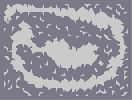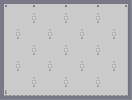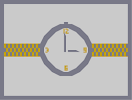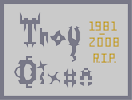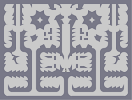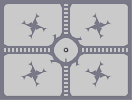The cave of Unforgettable Souls Rockets!!! 9 in the after noon In Memory of Troy Dixon I'm Back Baby! Just Yourself

Pages: (0)

### Di

FungShwaiMaChingChangChowQinShiHuangDi.

:)

### i only like

the white square in the middle.
it's so rebellious
my head! IT HURTS!